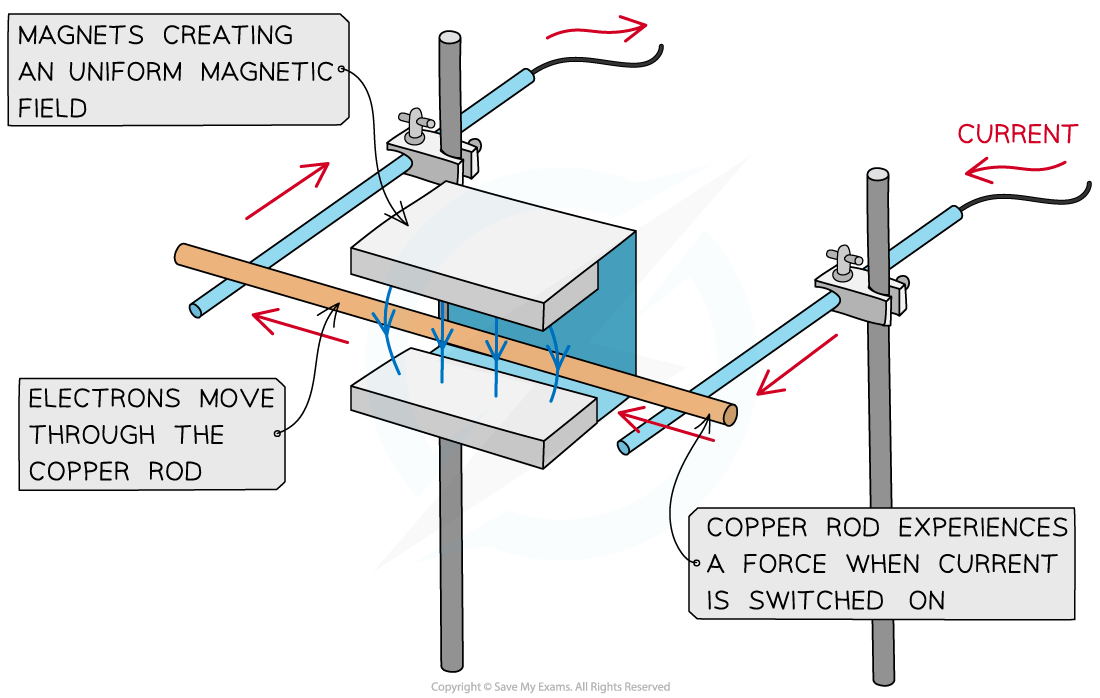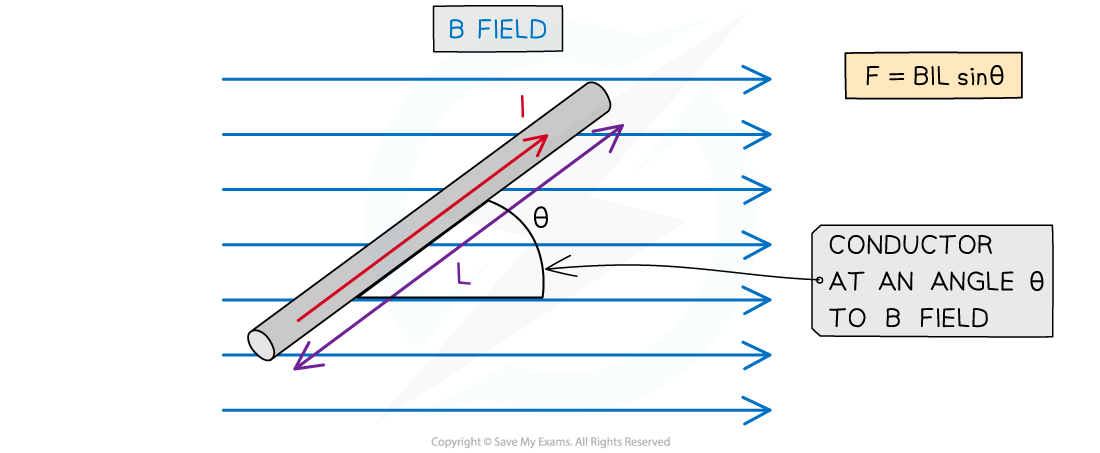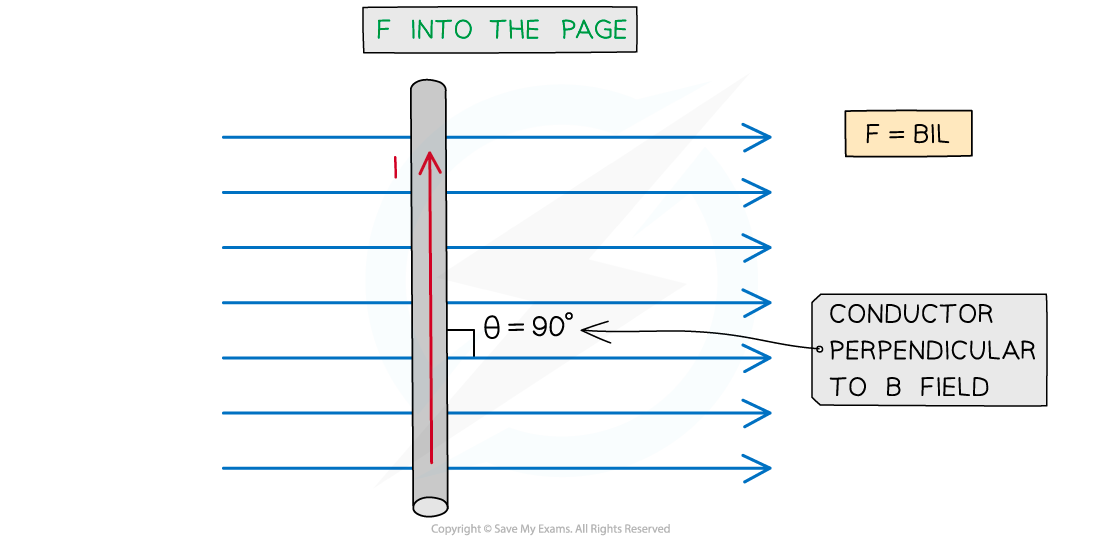# CIE A Level Physics复习笔记20.1.2 Force on a Current-Carrying Conductor

### Force on a Current-Carrying Conductor

• A current-carrying conductor produces its own magnetic field
• When interacting with an external magnetic field, it will experience a force
• A current-carrying conductor will only experience a force if the current through it is perpendicular to the direction of the magnetic field lines
• A simple situation would be a copper rod placed within a uniform magnetic field
• When current is passed through the copper rod, it experiences a force which makes it moveA copper rod moves within a magnetic field when current is passed through it

### Calculating Magnetic Force on a Current-Carrying Conductor

• The strength of a magnetic field is known as the magnetic flux density, B
• This is also known as the magnetic field strength
• It is measured in units of Tesla (T)
• The force F on a conductor carrying current I at right angles to a magnetic field with flux density B is defined by the equation

F = BIL sinθ

• Where:
• F = force on a current carrying conductor in a B field (N)
• B = magnetic flux density of external B field (T)
• I = current in the conductor (A)
• L = length of the conductor (m)
• θ = angle between the conductor and external B field (degrees)
• This equation shows that the greater the current or the magnetic field strength, the greater the force on the conductorMagnitude of the force on a current carrying conductor depends on the angle of the conductor to the external B field

• The maximum force occurs when sin θ = 1
• This means θ = 90o and the conductor is perpendicular to the B field
• This equation for the magnetic force now becomes:

F = BIL

• The minimum force (0) is when sin θ = 0
• This means θ = 0and the conductor is parallel to the B field
• It is important to note that a current-carrying conductor will experience no force if the current in the conductor is parallel to the field

#### Worked Example

A current of 0.87 A flows in a wire of length 1.4 m placed at 30o to a magnetic field of flux density 80 mT.Calculate the force on the wire.

Step 1: Write down the known quantities

Magnetic flux density, B = 80 mT = 80 × 10-3 T

Current, I = 0.87 A

Length of wire, L = 1.4 m

Angle between the wire and the magnetic field, θ = 30o

Step 2: Write down the equation for force on a current-carrying conductor

F = BIL sinθ

Step 3: Substitute in values and calculate

F =  (80 × 10-3) × (0.87) × (1.4) × sin(30) = 0.04872 = 0.049 N (2 s.f)

#### Exam Tip

Remember that the direction of current flow is the flow of positive charge (positive to negative), and this is in the opposite direction to the flow of electrons